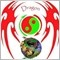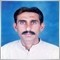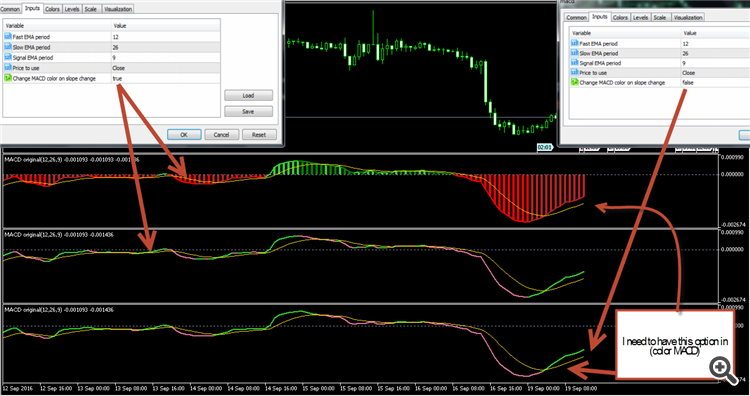# Elite indicators - metatrader 5 version :) - page 40177583

Dragon:

Dear Sir Thank you for the clarification.

See this picture, which painted dots what the role of the Yellow Line.  Please, all four colors appear with penetrating yellow line and match the original index

See the values

It is a long known fact that built in macd is using SMA for signal line while the original has to use EMA (as described in the link you have been provided). So, you are trying to compare things that can not be compared206

See the values

It is a long known fact that built in macd is using SMA for signal line while the original has to use EMA (as described in the link you have been provided). So, you are trying to compare things that can not be compared

Sir, this is a values177583

Dragon:

Sir, this is a values

Please, the values of macd and signal line, not the parameters206

:
Please, the values of macd and signal line, not the parameters
//+------------------------------------------------------------------+
//|                                                         MACD.mq5 |
//|                        Copyright 2009, MetaQuotes Software Corp. |
//|                                              http://www.mql5.com |
//+------------------------------------------------------------------+
#property copyright   "2009, MetaQuotes Software Corp."
#property description "Moving Average Convergence/Divergence"
#include <MovingAverages.mqh>
//--- indicator settings
#property indicator_separate_window
#property indicator_buffers 4
#property indicator_plots   2
#property indicator_type1   DRAW_HISTOGRAM
#property indicator_type2   DRAW_LINE
#property indicator_color1  Silver
#property indicator_color2  Red
#property indicator_width1  2
#property indicator_width2  1
#property indicator_label1  "MACD"
#property indicator_label2  "Signal"
//--- input parameters
input int                InpFastEMA=12;               // Fast EMA period
input int                InpSlowEMA=26;               // Slow EMA period
input int                InpSignalSMA=9;              // Signal SMA period
input ENUM_APPLIED_PRICE InpAppliedPrice=PRICE_CLOSE; // Applied price
//--- indicator buffers
double                   ExtMacdBuffer[];
double                   ExtSignalBuffer[];
double                   ExtFastMaBuffer[];
double                   ExtSlowMaBuffer[];
//--- MA handles
int                      ExtFastMaHandle;
int                      ExtSlowMaHandle;
//+------------------------------------------------------------------+
//| Custom indicator initialization function                         |
//+------------------------------------------------------------------+
void OnInit()
{
//--- indicator buffers mapping
SetIndexBuffer(0,ExtMacdBuffer,INDICATOR_DATA);
SetIndexBuffer(1,ExtSignalBuffer,INDICATOR_DATA);
SetIndexBuffer(2,ExtFastMaBuffer,INDICATOR_CALCULATIONS);
SetIndexBuffer(3,ExtSlowMaBuffer,INDICATOR_CALCULATIONS);
//--- sets first bar from what index will be drawn
PlotIndexSetInteger(1,PLOT_DRAW_BEGIN,InpSignalSMA-1);
//--- name for Dindicator subwindow label
IndicatorSetString(INDICATOR_SHORTNAME,"MACD("+string(InpFastEMA)+","+string(InpSlowEMA)+","+string(InpSignalSMA)+")");
//--- get MA handles
ExtFastMaHandle=iMA(NULL,0,InpFastEMA,0,MODE_EMA,InpAppliedPrice);
ExtSlowMaHandle=iMA(NULL,0,InpSlowEMA,0,MODE_EMA,InpAppliedPrice);
//--- initialization done
}
//+------------------------------------------------------------------+
//| Moving Averages Convergence/Divergence                           |
//+------------------------------------------------------------------+
int OnCalculate(const int rates_total,
const int prev_calculated,
const datetime &time[],
const double &open[],
const double &high[],
const double &low[],
const double &close[],
const long &tick_volume[],
const long &volume[],
{
//--- check for data
if(rates_total<InpSignalSMA)
return(0);
//--- not all data may be calculated
int calculated=BarsCalculated(ExtFastMaHandle);
if(calculated<rates_total)
{
Print("Not all data of ExtFastMaHandle is calculated (",calculated,"bars ). Error",GetLastError());
return(0);
}
calculated=BarsCalculated(ExtSlowMaHandle);
if(calculated<rates_total)
{
Print("Not all data of ExtSlowMaHandle is calculated (",calculated,"bars ). Error",GetLastError());
return(0);
}
//--- we can copy not all data
int to_copy;
if(prev_calculated>rates_total || prev_calculated<0) to_copy=rates_total;
else
{
to_copy=rates_total-prev_calculated;
if(prev_calculated>0) to_copy++;
}
//--- get Fast EMA buffer
if(IsStopped()) return(0); //Checking for stop flag
if(CopyBuffer(ExtFastMaHandle,0,0,to_copy,ExtFastMaBuffer)<=0)
{
Print("Getting fast EMA is failed! Error",GetLastError());
return(0);
}
//--- get SlowSMA buffer
if(IsStopped()) return(0); //Checking for stop flag
if(CopyBuffer(ExtSlowMaHandle,0,0,to_copy,ExtSlowMaBuffer)<=0)
{
Print("Getting slow SMA is failed! Error",GetLastError());
return(0);
}
//---
int limit;
if(prev_calculated==0)
limit=0;
else limit=prev_calculated-1;
//--- calculate MACD
for(int i=limit;i<rates_total && !IsStopped();i++)
ExtMacdBuffer[i]=ExtFastMaBuffer[i]-ExtSlowMaBuffer[i];
//--- calculate Signal
SimpleMAOnBuffer(rates_total,prev_calculated,0,InpSignalSMA,ExtMacdBuffer,ExtSignalBuffer);
//--- OnCalculate done. Return new prev_calculated.
return(rates_total);
}
//+------------------------------------------------------------------+177583

Dragon

At your picture, after the names of the indicators, there are some numbers.

Those are the values. Compare those values (not the mql code either)206

Dragon

At your picture, after the names of the indicators, there are some numbers.

Those are the values. Compare those values (not the mql code either)

There have two options of conversion.

The default option, which I want them to work with four colors.

//------------------------------------------------------------------

//------------------------------------------------------------------

#property indicator_separate_window

#property indicator_buffers 3

#property indicator_plots   2

#property indicator_label1  "MACD"

#property indicator_type1   DRAW_COLOR_LINE

#property indicator_color1  LimeGreen,PaleVioletRed

#property indicator_width1  2

#property indicator_label2  "Signal"

#property indicator_type2   DRAW_LINE

#property indicator_color2  Gold

//

//

//

//

//

enum enPrices

{

pr_close,      // Close

pr_open,       // Open

pr_high,       // High

pr_low,        // Low

pr_median,     // Median

pr_typical,    // Typical

pr_weighted,   // Weighted

pr_haclose,    // Heiken ashi close

pr_haopen ,    // Heiken ashi open

pr_hahigh,     // Heiken ashi high

pr_halow,      // Heiken ashi low

pr_hamedian,   // Heiken ashi median

pr_hatypical,  // Heiken ashi typical

pr_haweighted, // Heiken ashi weighted

pr_haaverage   // Heiken ashi average

};

input int      InpFastEMA      = 12;       // Fast EMA period

input int      InpSlowEMA      = 26;       // Slow EMA period

input int      InpSignalEMA    =  9;       // Signal EMA period

input enPrices InpAppliedPrice = pr_close; // Price to use

input bool     ColorOnSlope    = false;    // Change MACD color on slope change

//

//

//

//

//

double  macd[];

double  macdColor[];

double  signal[];

//------------------------------------------------------------------

//

//------------------------------------------------------------------

//

//

//

//

//

void OnInit()

{

SetIndexBuffer(0,macd     ,INDICATOR_DATA);

SetIndexBuffer(1,macdColor,INDICATOR_COLOR_INDEX);

SetIndexBuffer(2,signal   ,INDICATOR_DATA);

IndicatorSetString(INDICATOR_SHORTNAME,"MACD original("+string(InpFastEMA)+","+string(InpSlowEMA)+","+string(InpSignalEMA)+")");

}

//------------------------------------------------------------------

//

//------------------------------------------------------------------

//

//

//

//

//

double emas[];

#define _fast 0

#define _slow 1

int OnCalculate(const int rates_total,

const int prev_calculated,

const datetime& time[],

const double& open[],

const double& high[],

const double& low[],

const double& close[],

const long& tick_volume[],

const long& volume[],

{

if (ArrayRange(emas,0)!=rates_total) ArrayResize(emas,rates_total);

//

//

//

//

//

double alphafa = 2.0 / (1.0 +InpFastEMA);

double alphasl = 2.0 / (1.0 +InpSlowEMA);

double alphasi = 2.0 / (1.0 +InpSignalEMA);

for (int i=(int)MathMax(prev_calculated-1,0); i<rates_total; i++)

{

double price = getPrice(InpAppliedPrice,open,close,high,low,i,rates_total);

if (i==0)

{

emas[i][_fast] = price;

emas[i][_slow] = price;

macd[i]   = 0;

signal[i] = 0;

continue;

}

//

//

//

//

//

emas[i][_fast] = emas[i-1][_fast]+alphafa*(price-emas[i-1][_fast]);

emas[i][_slow] = emas[i-1][_slow]+alphasl*(price-emas[i-1][_slow]);

macd[i]   = emas[i][_fast]-emas[i][_slow];

signal[i] = signal[i-1]+alphasi*(macd[i]-signal[i-1]);

macdColor[i] = macdColor[i-1];

if (ColorOnSlope)

{

if (macd[i]>macd[i-1]) macdColor[i] = 0;

if (macd[i]<macd[i-1]) macdColor[i] = 1;

}

else

{

if (macd[i]>signal[i]) macdColor[i] = 0;

if (macd[i]<signal[i]) macdColor[i] = 1;

}

}

//

//

//

//

//

return(rates_total);

}

//------------------------------------------------------------------

//

//------------------------------------------------------------------

//

//

//

//

//

double workHa[];

double getPrice(enPrices pricet, const double& open[], const double& close[], const double& high[], const double& low[], int i, int bars)

{

//

//

//

//

//

if (pricet>=pr_haclose && pricet<=pr_haaverage)

{

if (ArrayRange(workHa,0)!= bars) ArrayResize(workHa,bars);

//

//

//

//

//

double haOpen;

if (i>0)

haOpen  = (workHa[i-1] + workHa[i-1])/2.0;

else   haOpen  = open[i]+close[i];

double haClose = (open[i] + high[i] + low[i] + close[i]) / 4.0;

double haHigh  = MathMax(high[i], MathMax(haOpen,haClose));

double haLow   = MathMin(low[i] , MathMin(haOpen,haClose));

if(haOpen  <haClose) { workHa[i] = haLow;  workHa[i] = haHigh; }

else                 { workHa[i] = haHigh; workHa[i] = haLow;  }

workHa[i] = haOpen;

workHa[i] = haClose;

//

//

//

//

//

switch (pricet)

{

case pr_haclose:     return(haClose);

case pr_haopen:      return(haOpen);

case pr_hahigh:      return(haHigh);

case pr_halow:       return(haLow);

case pr_hamedian:    return((haHigh+haLow)/2.0);

case pr_hatypical:   return((haHigh+haLow+haClose)/3.0);

case pr_haweighted:  return((haHigh+haLow+haClose+haClose)/4.0);

case pr_haaverage:   return((haHigh+haLow+haClose+haOpen)/4.0);

}

}

//

//

//

//

//

switch (pricet)

{

case pr_close:     return(close[i]);

case pr_open:      return(open[i]);

case pr_high:      return(high[i]);

case pr_low:       return(low[i]);

case pr_median:    return((high[i]+low[i])/2.0);

case pr_typical:   return((high[i]+low[i]+close[i])/3.0);

case pr_weighted:  return((high[i]+low[i]+close[i]+close[i])/4.0);

}

return(0);

}4691

Dragon:

There have two options of conversion.

The default option, which I want them to work with four colors.

Dragon

Man,is it not possible for you to keep clean this thread for what it was started,i means for Elite indicators MT5 version only and shift your problem,matter or issue to related thread for example request and idea or coding help ..... you have to know,boss dislike variant and congested topics in unrelated thread.

regards177583

dragon,

Which exact macd are you trying to duplicate and why?206

dragon,

Which exact macd are you trying to duplicate and why?

Dear Sir Yes it is MACD .

You need to work with a couple of options.

MACD works with four colors .

But made it work one option.206

mntiwana:

Dragon

Man,is it not possible for you to keep clean this thread for what it was started,i means for Elite indicators MT5 version only and shift your problem,matter or issue to related thread for example request and idea or coding help ..... you have to know,boss dislike variant and congested topics in unrelated thread.

regards

Dear just tell you the words of one here is not the arena for the show muscle.